﻿ MechDesigner Reference & User Interface > Dialogs > Dialog: Function-Block: Polynomial-Fit

# Dialog: Function-Block: Polynomial-Fit

Navigation:  MechDesigner Reference & User Interface > Dialogs >

# Dialog: Function-Block: Polynomial-Fit

## Polynomial-Fit FB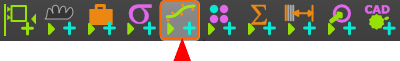The Polynomial Fit FB finds a concatenated series of Polynomials (5th order) that are approximately equal to the data at its input-connector. After you do a Polynomial-Fit, you can: •Transfer Polynomials directly to MotionDesigner as a new Motion name-tab. •Connect a wire from the output-connector of the Polynomial-Fit FB to the input-connector of a different FB. •Export the motion-values that you calculate as a text-file. •Export the values for position, velocity, and acceleration at the beginning and end of each polynomial segment as a CSV file. •Export the polynomials segments in a format suitable for the Schneider-Electric® EPAS4 servo-controller.

#### How to open the Polynomial-Fit FB dialog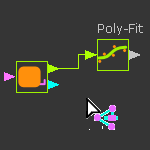To open the Polynomial-Fit dialog

 1.Double-click the Polynomial-Fit FB in the graphic-area OR 1.

The Polynomial-Fit dialog is now open.

### Polynomial-Fit dialog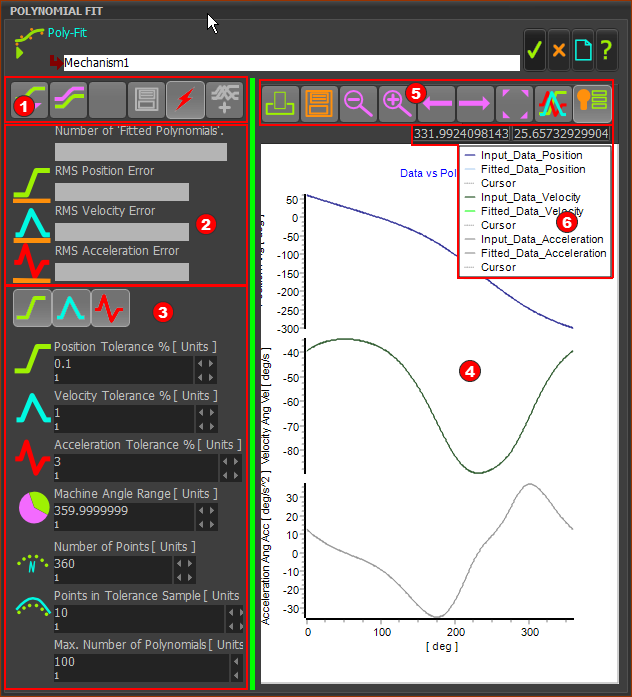Polynomial-Fit FB dialog/interface.

The Polynomial-Fit dialog: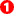Acquire and Save Toolbar - acquire and save results.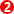Analysis of Results - RMS errors and the number of Polynomials.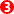Settings for Polynomial Fitting Algorithms - tolerances, limits, and range for polynomial fitting.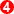Graph Plots: for the input-data and the calculated data from the Polynomials.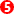Graph toolbar - view and save the graph-data in different ways.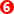Graph Legend. - graph plot color scheme for original data and fitted Polynomials, for each motion-derivative

####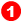Acquire and Save toolbar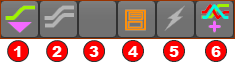Acquire and Save Results toolbar

The toolbar buttons are:Acquire (Capture, Get One Cycle) : Click this button to acquire data at the input-connector for one machine-cycle - this is the Raw-Data.Fit Polynomials to Data : Click to Fit Polynomials to the Raw-DataStop Algorithms : (see also Auto-Run) The algorithms usually fit polynomials within a few seconds. If you do not see the results after a few seconds, then click this button to stop the algorithms.Save Coefficients : Click to save the results to a CSV file, or a EPAS4 file that is suitable for a Schneider Electric servo-controller.Auto-Run : Immediately allow the algorithms to find polynomials fit the data to the accuracy as specified, and with less or equal to the maximum number of polynomials.Send Polynomials to MotionDesigner : Add a new Motion name-tab, and send the Polynomials as a new motion.

####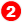Analysis of Results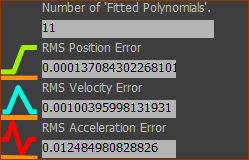Number of Fitted Polynomials in Fit (read-only) The number of Polynomials in the numerical solution. See also: Maximum Polynomial Count. in the Settings for Polynomial Fitting Algorithms. RMS Position Error, RMS Velocity Error, RMS Acceleration Error (read-only). They give the RMS (Root, Mean, Square) error for each motion-derivative between the Polynomial-Fit and the data at the input-connector as a percentage.

####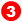Settings for Polynomial Fitting Algorithms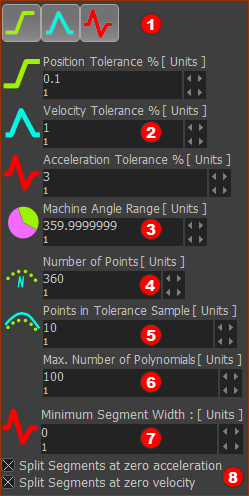Parameters to enter before you click Fit Polynomials to Data (buttonin the Acquire and Save toolbar, above)Position, Velocity, Acceleration buttons. Click each button to identify the motion-derivatives to which you want to Fit Polynomials.Position, Velocity, Acceleration Tolerance % Enter the tolerance, as a %, for each motion-derivative, within which the Fitted Polynomials should represent the data at the input-connector.Machine Angle Range Optionally, reduce the range for which you want to calculate PolynomialsNumber of Points The number of data points that are calculated from the Polynomials, to plot the graphs.Point in Tolerance Sample of Points The number of points that the algorithm uses to fit each polynomial to the data at the input-connector.Maximum number of Polynomials. Upper limit of the number of Fitted-Polynomials in the  data.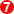Minimum Segment Width. Lower Limit for the width of a Segment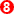Split Segment at Zero Acceleration / Velocity check-boxes Force the solutions to split the motion (add a new Segment) at which the Acceleration / Velocity crosses zero - positive to negative and vice versa.

####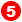Graph toolbar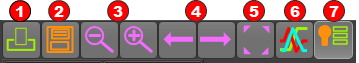The toolbar buttons are:Print Graphs :  Print the graphs immediately to the default printerSave Graph Data: Save the Data to a text file.Zoom Out and Zoom In to the Graph DetailsPan Left and Pan Right : Click to save the results to a CSV file, or a EPAS4 file that is suitable for a Schneider-Electric servo-controller.Zoom ExtentsToggle display: Plot three separate graphs OR Plot three stacked on-top of each otherToggle display:  Do show Graph Legend OR Do NOT show Graph Legend

####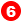Graph Legend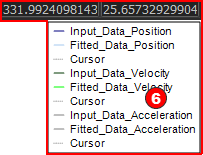Graph plot color scheme for •original, or input, data •fitted data calculated from the fitted polynomials •each motion-derivative •Cursor - you can click ON a graph of each motion-derivative to show a cursor. The X-Y data-values of the active cursor is above the graphs.